Journal of Civil, Construction and Environmental Engineering
Volume 1, Issue 1, December 2016, Pages: 1-11

The Application of Graphical Method to Create Sandy Soils with Given Physic-Mechanical Properties

Korolev V. A., Zhang Shengrong

Department of Engineering and Ecological Geology, Moscow State University Named M.V., Lomonosov, Russia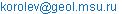(Korolev V. A.)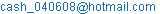(Zhang Shengrong)

Korolev V. A., Zhang Shengrong. The Application of Graphical Method to Create Sandy Soils with Given Physic-Mechanical Properties. Journal of Civil, Construction and Environmental Engineering. Vol. 1, No. 1, 2016, pp. 1-11. doi: 10.11648/j.jccee.20160101.11

Received: October 3, 2016; Accepted: October 14, 2016; Published: November 19, 2016

Abstract: In the article we mainly discussed how to adopt graphical method to show the influence of particle size on the mechanical properties of mixed soils. Based on the extensive experimental data, using triangle graphics, as well as software Matlab, we could describe and model the change of the mechanical properties of mixed soils. Through this method, we can create the soil with the needed requirements, and this will be very helpful in construction.

Keywords: Sand, Granulometric Composition, Triangular Diagram, Density, Porosity, Angle of Internal Friction, Optimum Mixture

1. Introduction

Sandy and coarse clastic soils are considered as important materials in the road and engineering constructions. However, the natural sandy and macro-fragmental soils generally don’t have high quantities of density. In addition, due to the different characteristics of various sandy and macro-fragmental soils, the individual natural sand and coarse clastic soils often do not satisfy the properties of complex engineering conditions. Therefore, in practice people often use different ways to improve the properties of the soil by artificial regulation granulometric composition.

Currently, in order to improve the properties of created artificial dispersed soils, most research work aimed at exploring the various factors, which can affect their properties. Among them, there are granulometric composition, soil density, mineral composition, moisture content and so on. The leading factor for sandy and coarse clastic soils is their granulometric compositions.

By now the influence of granulometric composition on the mechanical properties of soil mixtures has been studied various scientists [1,2,4,13], for example, V.V. Ohotin , N.N. Ivanov , A.N. Elmolaeva , Luis E. Vallejo [9,10] and so on.

In the view of N.N. Ivanov , friction resistance of soil mixtures is mainly due to the size of particles, their mineral skeleton, homogenous, form and the degree of roundness particles.

However, the question of creation sandy or coarse clastic soils with predetermined physic - mechanical properties remains to be resolved until now. Then our missions are formulated as follows: there are a number of granulometric fractions (or a series of soils with predetermined granulometric compositions) with predetermined physic - mechanical properties (the angle of internal friction, the modulus of total deformation); to be required to determine that, in order to obtain the soil mixture with the required (for example, maximum) strength in dense disposition, in which proportion these factions should be mixed?

Study of this question was focused on our work.

2. The Object of Research

Soils, of which the various granulometric fractions were selected, with different granulometric composition have been chosen for our researches. The original natural sandy soils were alluvial or fluvial formations. From them fractions with different sizes (1-2, 0,5-1, 0,25-0,5 и 0,1-0,25 mm) could be distinguished by screening.

Laboratory method for measuring the mechanical properties of original fractions and soil mixtures was based on standard methods [5,8] and consisted of the following.

The angle of internal friction (φ) was determined by device VSV-25. Testing each sample (mixture) was conducted according to the plane shear test under vertical loadings 0.1, 0.15 and 0.2 MPа. The original selected fractions are loaded into the device VSV-25 in dense disposition. The obtained results of angle of internal friction are presented in the Tab. 1.

Tab. 1. The angle of internal friction of the studied fractions in dense disposition.

 Size of fractions, (mm) tgφ φ, (o) 2-1 1.3 53 1-0.5 1.09 47 0.5 - 0.25 0.95 44 0.25-0.1 1.01 45 <0.1 0.87 41

In order to assess the modulus of total deformation (Еtot), we used their standard (normative) values (E, MPa) for sandy soils of Quaternary deposits according to Snip2.02.01-83 (Building Codes and Regulations) . The values of E were determined from the known values of the mixture’s porosity (n). The obtained data are presented in table 2.

Tab. 2. Standard values of the modulus of total deformation of the studied fractions in dense disposition.

 Parameter Size of fractions, (mm) 1 - 2 0,5 – 1 0,25–0,5 0,1–0, 25 <0,1 Porosityn, % 0.39 0.37 0.37 0.39 0.41 Modulus of total deformation Еtot, MPа 32 37 37 30 12

3. The Modeling Method of Soils with Given Properties

The modeling of mixtures with desired properties could be carried out by graphical method. Here we used 2 graphic ways.

The first method is the application of triangular diagram, which is a modified type of Ferre triangular. In contrast to Ferre triangle, the point within the triangle can represent not only the granulometric composition, but also the values of any parameter of physical or physic – mechanical properties of created soils (for example, the values of φ, E, etc.) (Fig. 1).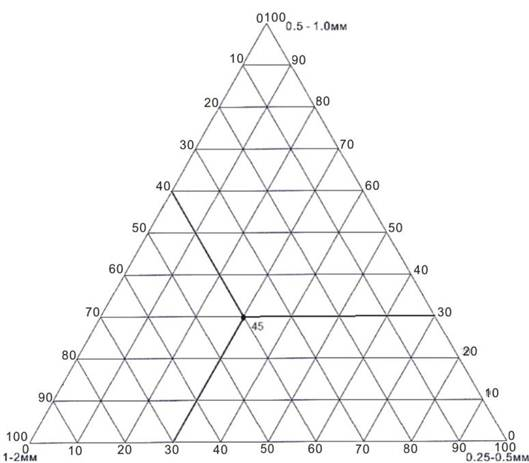Fig. 1. Thetriangle with the marked value of angle of internal friction (φ=45o) of mixture at a certain ratio of the analyzed fractions.

On the triangular diagram the points, which lie on the vertices of the triangle, represent 100% content of the original fractions, which constitute the granulometric mixture. And the point inside the triangle, which is marked with the number 45, reflects two values: granulometric composition and the value of angle of internal friction: first, it shows that the mixture formed by three fractions with mass ratio, respectively, equal to 4:3:3; secondly, it shows that the value of angle of internal friction of the mixture at this ratio is 45o.

Thus, similarly the values of angle of internal friction can be marked on the triangular diagram and for other points, reflecting the different proportions of granulometric fractions or granulometric composition of soil.

Combining the values of these points by contour, we can build a triangular graph representing the variation of this property (in our case - the angle of internal friction) in any quantitative ratio of the analyzed fractions. This method was developed and applied by us earlier .

In addition to this method, modeling of compounds could be performed by the MATLAB program, which can be used to build a visual 3D model of the changes of various properties of the created mixtures. These 3D models allow us directly and clearly to observe regularities of changing in any properties depending on the granulometric composition of the mixtures.

Properties of the created compounds are determined by their factions. Changing the quantity of any components of the fractions will cause a change in the properties of the created mixtures. The relationship between any properties of mixtures, which contain three factions, and content of their constituting fractions may be reflected by a generalized function of three variables:

A=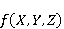(1)

Here, A - the value of any properties of mixtures (for example, E, φ, etc.); X, Y, Z– mass content of the component fractions.

Besides that, the values of X, Y, Z must satisfy the condition that their sum equals 100. From this It follows that only two fractions (X,Y,Z) are independent variables, and the third – dependent: its content is determined by the sum of the other two and can be found by their difference. Thus, the function A can be rewrote in this form:

A=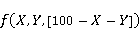(2)

In other words, a mixture consisted of n fractions only have (n-1) independent factions, or independent variables.

Based on this, it follows that, if the mass contents of any two component fractions have been known by us, then they will reflect the granulometric composition of the mixtures and their corresponding properties.

In other words, the properties of the created mixtures of the three factions can be reflected through any two original fractions. It allows differently to present graphical models of dependency А=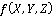.In particular it is possible to build three-dimensional or 3D models.

So, for example, three-dimensional models for mixtures consisted of three fractions, can be designed in this way. The Y-axis of three-dimensional models indicates the values A of any properties of mixtures (e.g., dry density (ρd), E, φ, etc.). The other two coordinate reflect the content of any two components of the fractions in percent (X, Y or Z). In our case, in order to better compare the influence of aggregates with the smallest size, we putted the values of the content of larger factions into the X-axis and Y-axis.

4. Results

4.1. The Results of Soils Modeling with Desired Deformation Properties

Now, we will turn to the results of our study and discuss the regularities of variation in the modulus of total deformation (Etot.). The results are shown in Fig. 2.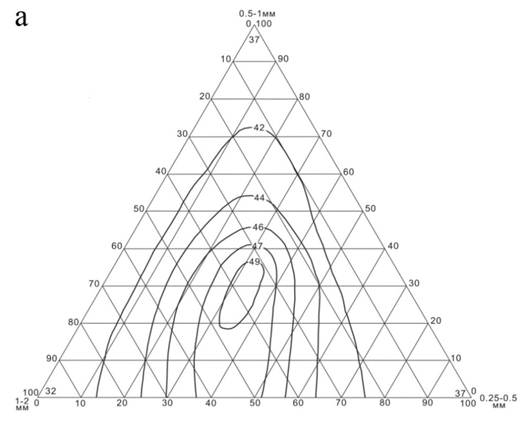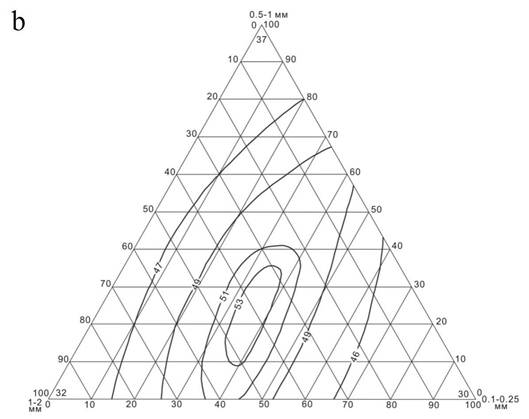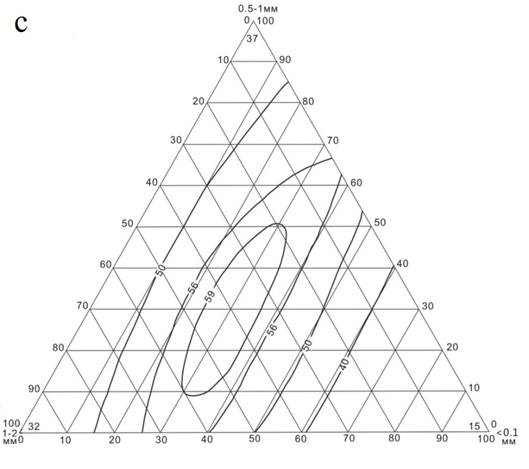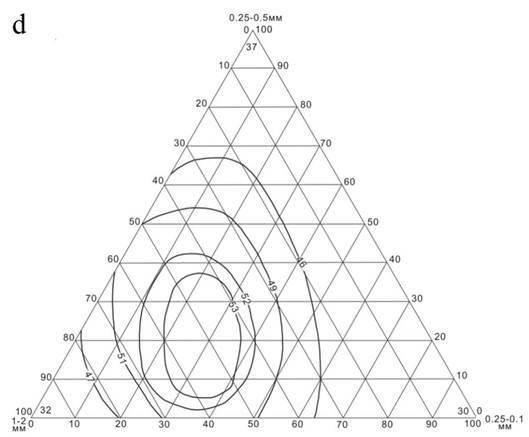Fig. 2. Triangular diagrams of the variations of the normative values of modulus of total deformation (Etot., MPa) mixtures consisted of three fractions: a – 1-2 mm, 0.5-1 mm and 0.25-0.5 mm; b - 1-2 mm, 0.5-1 mm and 0.25 to 0.1 mm; c - 1-2 mm, 0.5-1 mm, <0.1 mm; d - 1-2 mm, 0.25-0.5 mm and 0.1-0.25 mm.

As can be seen from the obtained data, the contours with the same normative values of the modulus of total deformation (Etot.) on the triangular diagram are located mainly central-symmetrically: the maximum values of the modulus of total deformation (Etot) of soils consisted of three fractions are close to the center. Thus, maximum values on the chart (Etot.) slightly displaced down and to the left, i.e. in the direction of increasing the largest fraction 1-2 mm.

For mixtures of two fractions, the values Etot (on the sides of the triangle in Fig. 2) are affected by the diametric ratio of the used fractions. If the ratio of two used fractions (dmax/dmin) is not more than 2:1, then the dry density (ρd) of mixture practically doesn’t change. On the triangular diagram it is reflected that the values on the sides of triangle (Etot) remains constant.

Besides that, from the analysis of the triangular diagrams in Fig. 2 we can draw the conclusion that when two aggregates were added to the monodisperse coarse – grained soil, aggregate with minimal particle size plays a major role. Values of Etot will increase with the decrease in the minimum diameter of the fractions. In our case, when the minimum particle size (dmin) of fractions less than 0.1 mm, the values of the modulus of total deformation (Etot) will obtain the maximum value which equals to 59 MPa. And when dmin = 0.25-0.5 mm, the maximum value of Etot will be reduced to 53 MPa.

When we add a smaller fraction to other two constituent fractions with a constant ratio of mass, the values of the modulus of total deformation (Etot) gradually will increase to a maximum value.

For mixtures consisted of three fractions, we found that the maximum value of modulus of total deformation (Etot) will be obtained if the mass content of each subsequent fraction refers to previous approximately 3:7 (see the contour lines inside the chart in Fig. 2).

With the help of software MATLAB we could build three-dimensional (3D) models (Fig. 3) for mixtures of three factions with different mass relations. It is possible to directly observe the regulative of changes in the values of the modulus of total deformation.

On the figures the area, which is painted redder, characterize the higher normative values of the modulus of total deformation (Etot). It has been seen that the area closed to the central region has relatively higher values of the modulus of total deformation (Etot) than other area. It means that adding a certain amount of smaller particles to the other two mixtures contributes to improve the normative values of the modulus of total deformation (Etot).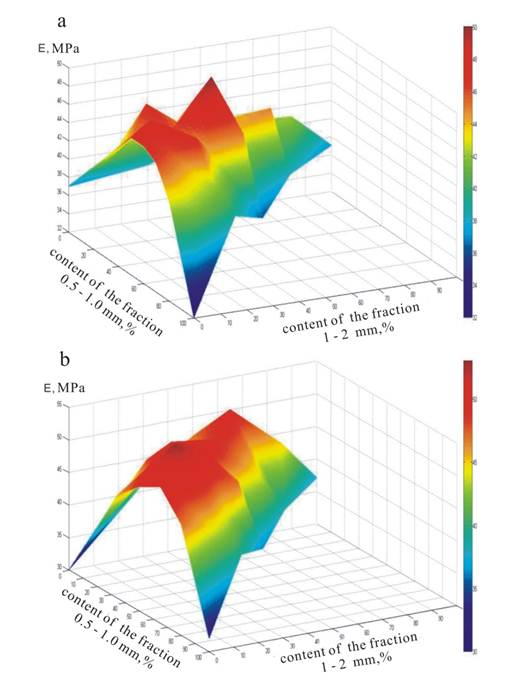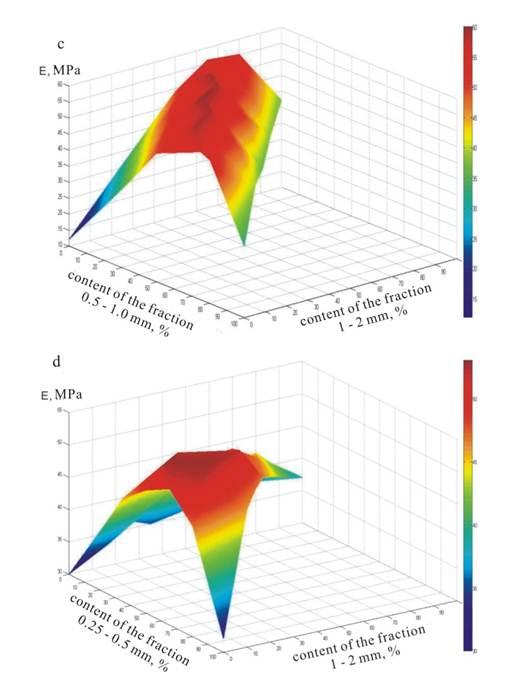Fig. 3. 3D model of change in normative values of the modulus of total deformation of created three faction mixtures: a – 1-2 mm, 0.5-1 mm and 0.25-0.5 mm; b - 1-2 mm, 0.5-1 mm and 0.25 to 0.1 mm; c - 1-2 mm, 0.5-1 mm and <0.1 mm; d - 1-2 mm, 0.25-0.5 mm and 0.1-0.25 mm.

4.2. The Results of Soils Modeling with Desired Strength Properties

First of all, we will discuss the easiest option – the mixture consisted of two fractions. The obtained results are shown in Fig. 4.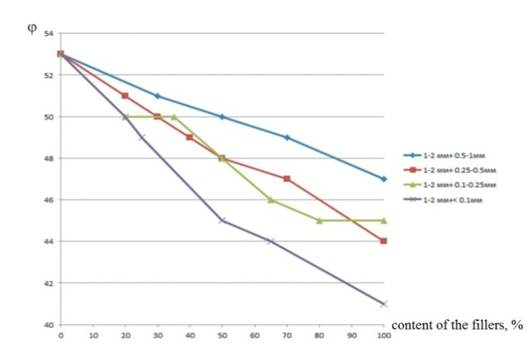Fig. 4. The regulative of change in the values of the angle of internal friction (φ) in the condition of adding different fillers to the fraction 1-2 mm.

From this figure we have seen that the values of angle of internal friction of mixtures consisted of two fractions; regularly decrease with decreasing content of the large particles (in our case 1-2 mm). If the size of the added particles becomes smaller, the impact on reducing the values of angle of internal friction created mixtures will be greater.

Besides that, Fig. 4 shows that the relationship between the values of angle of internal friction and the content of fraction 1-2 mm is closed to linear. Based on this, we can describe the relationship by regression analysis. The obtained results and the regression equation are shown as follows:

Soil mixtures consisted of the fractions 1 – 2 mm and 0,5 – 1,0 mm:

φ = -0,0586Х + 52,931,R = 0.9966

Soil mixtures consisted of the fractions 1 – 2 mm and 0,25 – 0,5 mm:

φ = -0,0876Х + 52,931,R = 0.9918

Soil mixtures consisted of the fractions 1 – 2 mm and 0,1 – 0,25 mm:

φ = -0,0841Х + 52,737,R = 0.9356

Soil mixtures consisted of the fractions 1 – 2 mm and 0,25 –,05 mm:

φ = -0,1211Х + 52,247,R = 0.9699,

here, Х – the content of the fillers; R - the coefficient of correlation.

We could see that the correlation coefficient R is more than 0.9 for all of the equations. As a result, we can say that in our study there is a close linear relationship between the values of angle of internal friction and the content of large particles.

And as for the modulus of total deformation, the same triangular diagrams were built to study the change in angle of internal friction of mixtures of three factions (Fig. 5).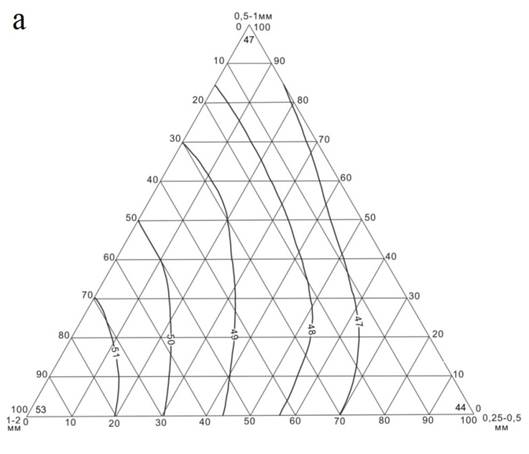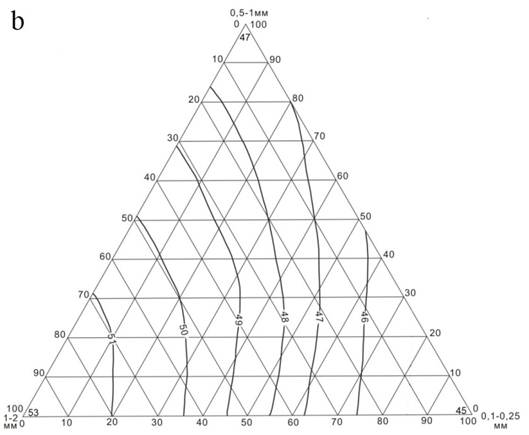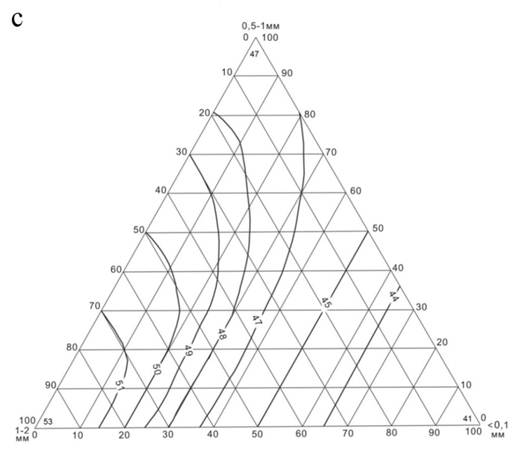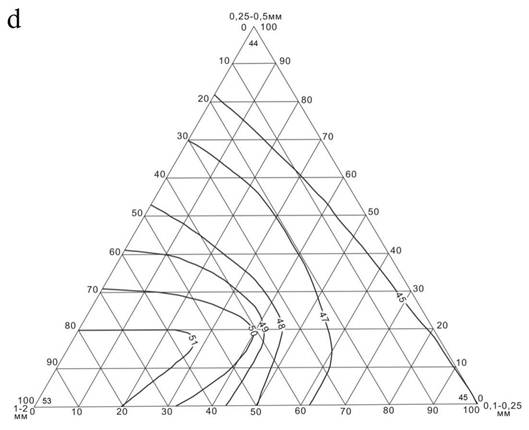Fig. 5. Triangular diagrams of change in the angle of internal friction of mixtures consisted of three fractions: a – 1-2 mm, 0.5-1 mm and 0.25-0.5 mm; b - 1-2 mm, 0.5-1 mm, and 0.25 to 0.1 mm; c - 1-2 mm, 0.5-1 mm, <0.1 mm; d - 1-2 mm, 0.25-0.5 mm and 0.1-0.25 mm.

From these figures it follows that in the mixture the concentration of large fractions (1-2 mm) proportionally determines the value of angle of internal friction (φ) of soil mixtures. It is because the larger particles generally have more acute-angled shape and are less rounded. This will lead to increase the hooking of neighboring particles for each other. In addition, in the dense condition the strength of the mixture is mainly influenced by the content of the large fraction, although too many large fractions lead to a slight decrease in the density of the skeleton of mixes.

Adding small factions to the large fractions at the initial stage apparently allows to increase the density of the skeleton, but at the same time leads to some reduction in the strength of mixturesreduce the value of φ). Moreover, the smaller the value of the angle of internal friction of the added particle, the sharper the change in the angle of internal friction created mixtures (Fig. 5 b and c).

We also built 3D models of change in angle of internal friction depending on the composition of mixtures (see Fig. 6). It indicates that, unlike the models for the modulus of total deformation, the models of angle of internal friction approximately have the characteristic of inclined planes. The maximum values of the angle of internal friction are not positioned in the center, and are concentrated in the outermost region. This means that the more the content of large particles in the soil mixtures, the greater their values of angle of internal friction.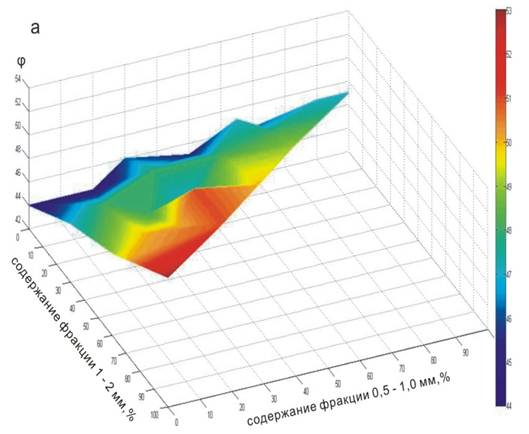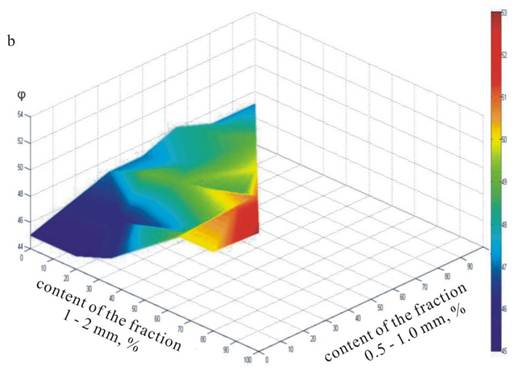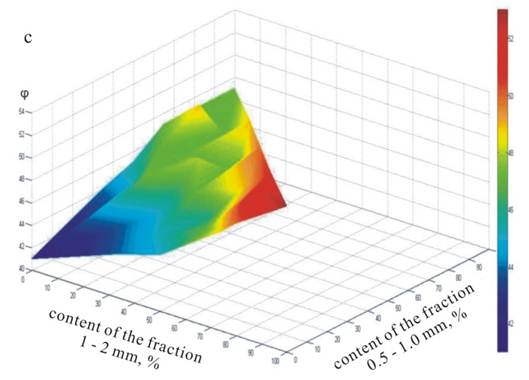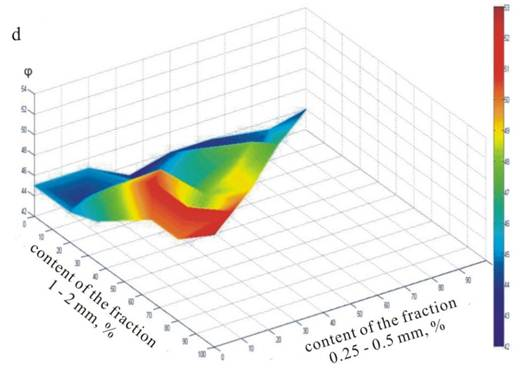Fig. 6. 3D models of the change in angle of internal friction created mixtures of three factions: a – 1-2 mm, 0.5-1 mm and 0.25-0.5 mm; b - 1-2 mm, 0.5-1 mm, and 0.25 to 0.1 mm; c - 1-2 mm, 0.5-1 mm, and <0.1 mm; d - 1-2 mm, 0.25-0.5 mm and 0.1-0.25 mm.

Of course, the values of angle of internal friction of the soil mixtures are determined not only by the content of coarse fraction, and by all the components of the original soil fractions. Based on this, we can ask: is there any mathematical relationship between the values of angle of internal friction of soil fractions and their constituents? If there is, what is the relationship?

In order to establish the answer to this question, we applied regression analysis. The results were showed as the following:

For soil mixture of 3 fractions 1 – 2 mm, 0,5 – 1,0 mm, 0,25 – 0,5 mm:

φ = 1,2948Х1- 2 + 1,2396Х0,5 - 1 + 1,208Х0,25- 0,5 – 76,499, R = 0.93306

For soil mixture of 3 fractions 1 – 2 mm, 0,5 – 1,0 mm, 0,1 – 0,25 mm:

φ = -0,78479Х1- 2 - 0,82298Х0,5 - 1 – 0,85617Х0,1- 0,25 + 130,31, R = 0.79522

For soil mixture of 3 fractions 1 – 2 mm, 0,5 – 1,0 mm, and < 0,1mm:

φ = 0,51512Х1- 2 + 0,4831Х0,5 - 1 + 0,41156Х< 0.1, R = 0.79473

For soil mixture of 3 fractions 1 – 2 mm, 0,25 – 0,5 mm and < 0,1mm:

φ = 1,0652Х1- 2 + 0,96953Х0,25- 0,5 + 0,97444Х< 0.1 – 53,302, R = 0.84405,

here, X1 - 2, X0,5 – 1, X0,25 - 0.5, X0,1 - 0.25, X< 0.1, the content of soil fractions, respectively, 1 - 2 mm, 0.5 - 1.0 mm, 0.25 – 0.5 mm, 0.1 - 0.25 mm and less than 0.1 mm.

From the equations above it follows that the soil mixtures consisted of soil fractions of 1-2 mm, 0.5-1.0 mm 0.25-0.5 mm and 1-2 mm, 0.25-0.5 mm and <0.1 mm have a relatively high coincidence with built linear models. In other words, these two soil mixtures have relatively well linear relationship between the values of angle of internal friction (φ) and the content of component of soil fractions.

And with decreasing the size of soil fractions, added to soil mixtures of 1-2 mm and 0.5-1.0 mm, values of R relatively decrease from 0,93306 to 0,79473. This is because adding fractions with smaller sizes can reduce the shearing strength of the created mixtures. As a result, the linear relationship between the values of angle of internal friction and the content of component soil fractions will be broken.

5. Conclusion

Thus, after discussing the obtained results, we can draw the following conclusions:

(1)    For the granulometric mixtures, consisted of three soil fractions: 1). The normative values of modulus of total deformation (Etot) of soil mixtures are mainly determined by the value of the ratio of diameters of the larger and smaller particles (dmax/dmin). The higher the ratio, the greater the value of modulus of deformation of created mixtures. 2) the maximum value of the modulus of total deformation (Etot) of the soil mixtures consisted of the three fractions will be obtained if the content of each subsequent fraction refers to previous approximately 3:7.

(2)    In the dense addition the strength of soil mixtures, measured by the angle of internal friction (φ), is mainly influenced by the content of the large fraction. The smaller the angle of internal friction of the added particles, the more change in the angle of internal friction of created soil mixtures.

(3)    The soil mixtures of two factions have a close correlation relationship between the values of angle of internal friction and the content of component fractions. The soil mixtures consisted of 3 fractions: 1 - 2 mm, 0.5-1.0 mm 0.25-0.5 mm and 1-2 mm, 0.25 – 0.5 mm and <0.1 mm have relatively well linear relationship between the values of angle of internal friction and the content of component fractions. Adding soil fractions with smaller sizes can reduce the shearing strength of the created soil mixtures and lead to disrupt the linear relationship between the values of angle of internal friction and the content of component soil fractions.

(4)    Three-dimensional (3D) models of the change in the deformation properties of the created soil mixtures have approximately the same form of curved surfaces. The highest values of the deformation properties are located in the central area of the 3D model. But for the strength properties of created soil mixtures, three-dimensional models approximately have the inclined planes. In this way the highest values of angle of internal friction are located in their extreme area.

References

1. Babenko V. A., Nikiforov N. V., Voznesenskiy E. A.Theoretical and methodicalaspects of experimentalresearchesof sand-clay filleraggregatecomposition and properties of coarse-grained soils // Engineering Geology. No. 5.2012.20-35p. (in Russian)
2. BabenkoV. A.,Funikova V. V.,VoznesenskiyE.A.Behaviourof lateral pressureincoarse-grained soils with different amounts ofafilleraggregatein true triaxialtest//GeotechnicalEngineering.№ 1-2.2014.66-73p. (in Russian)
3. Ermolaeva A. N. Experimental – analytical investigation and evaluation of mechanical properties of soils with coarse inclusions. //IzvestiyaVNIIG, Vol.235, 1999, 17-24p.(in Russian).
4. FengRuiling, Wang Yuan. The study of compatibility of clay soils with the content of clastic fractions.// Journal of Highway and Transportation Research and Development. Vol. 25, No. 9.Sep. 2008. 241-245 p.
5. GOST 12248-96. Methods of laboratory determination of strength and deformability characteristics. M.: IPK «Izdatel'stvostandartov», 2005. (in Russian).
6. Ivanov N. N., Puzakov N. A., TulaevA.Ya.,Andrulionis E. P. Construction of auto roads (in 2 parts) / [Edited by N.N. Ivanov]. M.: Transport, 1969. 469 p. (in Russian).
7. Korolev V.A., Zhang Shengrong.Modeling of sandy soils with specified physical and physical-mechanical properties. – Engineering Geology, 2015, № 4, pp.6-14. (in Russian).
8. Laboratory works on Soil Science/ [Edited by V. T. Trofimov, V. A. Korolev]. M.: Vysshayashkola, 2008. 519 p. (in Russian).
9. Luis E. Vallejo, Roger Mawby. Porosity influence on the shear strength of granular material–clay mixtures. //Engineering Geology 58 (2000) 125–136.
10. Luis E. Vallejo. Interpretation of the limits in shear strength inbinary granular mixtures. // Can. Geotech. J. Vol. 38, 2001. С. 1098 – 1104
11. Manual for designing the bases of buildings and structures (SNiP 2.02.01-83). - M.: Stroyizdat, 1986. 415 p.(in Russian).
12. Okhotin V. V. Soil Science. SPb.: ANTT-Print, 2008. 231p. (in Russian).
13. Zhang Zhifeng, etc. Test on mechanic properties of graded soil. // Journal of Chang'an University (Natural Scienct Edition).Vol.29, No. 4 Jul. 2009. 17-19 p.

 Contents 1. 2. 3. 4. 4.1. 4.2. 5.
Article ToolsAbstractPDF(797K)